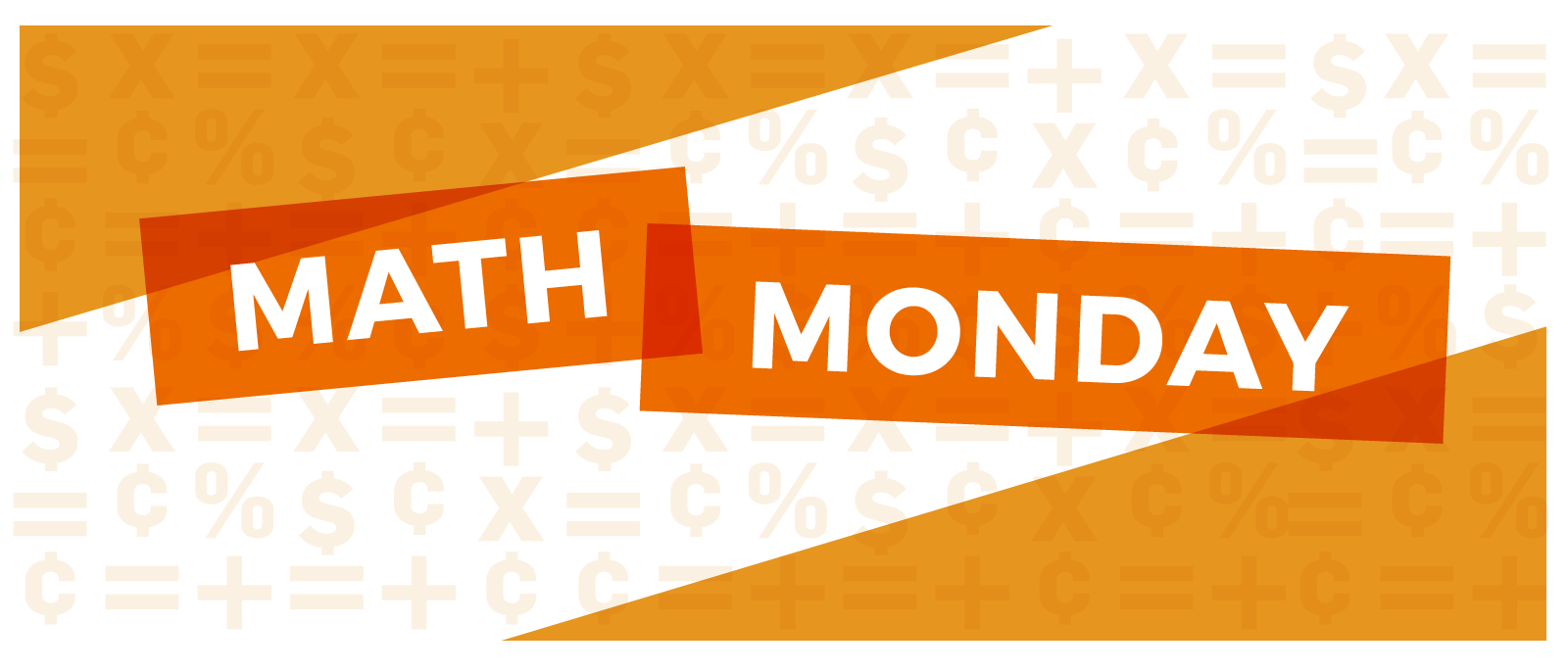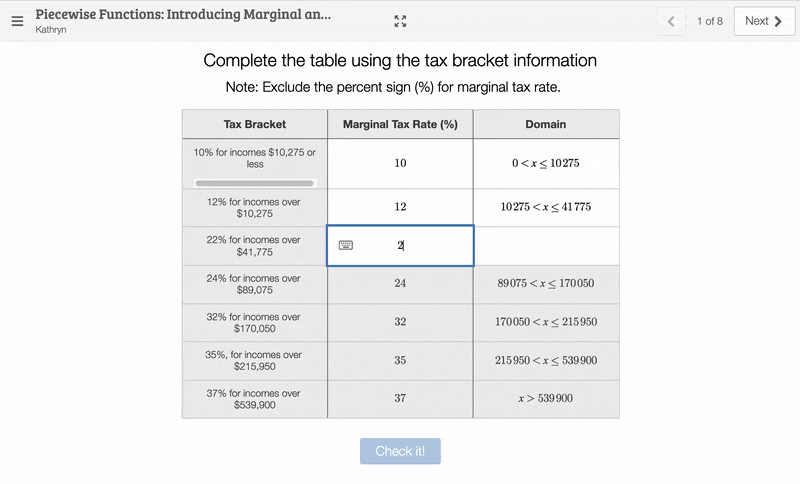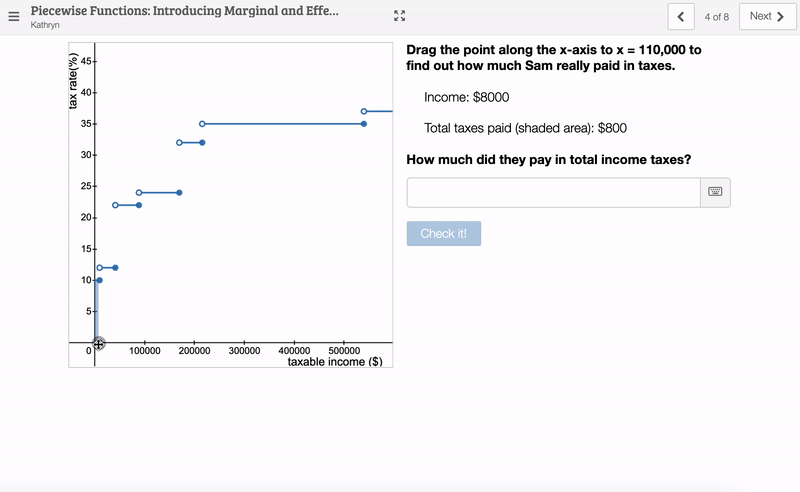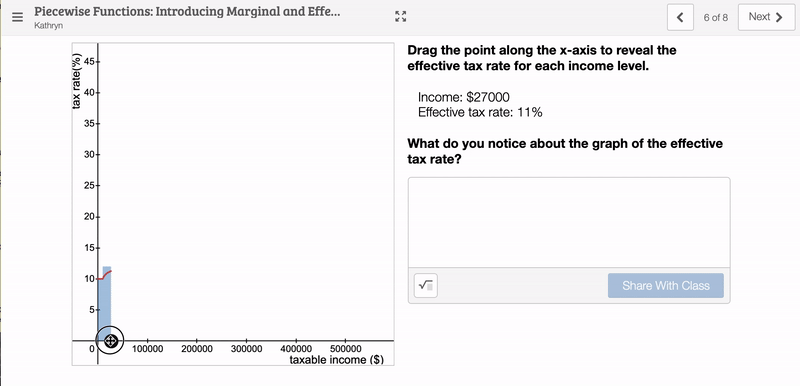Feb 13, 2022
Math

### Math Monday: Piecewise Functions - Introducing Marginal and Effective Tax Rates

This Math Monday, fall in love with Desmos as we explore the activity Piecewise Functions: Introducing Marginal and Effective Income Tax Rates

This Desmos activity corresponds to Level 1 of Application FA-1.7; it uses self-checking questions to practice writing equations for piecewise functions and using function notation. The interactive graph reinforces how income taxes are calculated using marginal tax rates and introduces students to effective tax rates.

Part I: Write the Piecewise Equation for Marginal Tax Rate

First, students complete the equation for the piecewise function representing marginal tax rate. They can self-check their answers and get feedback on which part of the equation they need to fix. As the teacher, you’ll see a warning if students check their work more than three times.Part II: Marginal Tax Rate Graph

Next, students see the stepped graph for the marginal income tax rate. They can interact with the graph by dragging a point along the x-axis. This interaction conceptually reinforces the way income taxes are calculated using tax brackets; the shaded area represents the total taxes paid.Students find Sam’s total taxes paid and calculate their effective tax rate. Then, students reflect on why Sam’s effective tax rate differs from their marginal tax rate.

Part III: Effective Tax Rate

Finally, students reveal the graph for the effective income tax rate as a function of taxable income. Again, the blue shading represents total taxes paid.One the following slide, students will make a prediction about the end behavior of this function: what is the highest possible effective income tax rate? Then, students can reveal the same graph, scaled to show higher x-values, and reflect on their prediction.

Where to Find NGPF’s Desmos Activities

If you’re using our Financial Algebra curriculum, you’ll find Desmos activities built directly into the Lesson Guides and Application Answer Keys.

To look through NGPF’s library of Desmos activities, you can check out our Financial Algebra Collection.

Want More?